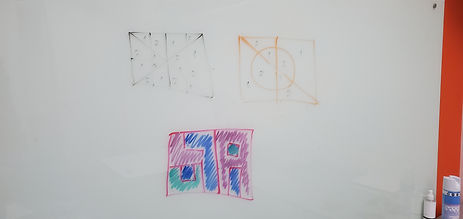## Mr. Jake

### Target 1​

###### Lesson Type:

Continuation

Number Operation

:

Number Meaning

Understand that quantities can be either positive or negative.

###### 1:

Understand that quantities having a value of more than zero are positive and that quantities having a value of less than zero are negative.

###### 2:

Understand that zero is neither positive nor negative.

###### 3:

Understand that positive and negative numbers occur in the real world.

###### 4:

Understand the relationship between positive and negative numbers.

###### 5:

Understand that integers get smaller in value as they move left in order, and larger as they move right in order.

6th

###### Vocabulary:

Positive, Negative

Activities:

Practiced solving addition and subtraction problems with positive and negative values### Home Exploration

###### Guiding Questions:## Absent Students:

Sahil

### Target 2

:

###### 1:

Use the standard algorithm for solving multi-digit division equations.

###### 2:

Understand the role of place-value in division strategies.

###### 3:

Understand that there can be a remainder in a division problem.

6th

###### Vocabulary:

Division, Divisible

Activities:

Studied the rules of division for numbers 1 through 9

Determined patterns that occur when dividing by the same number### Home Exploration

###### Guiding Questions:### Target 3

:

###### 1:

Look at a visual puzzle to analyze the pieces and determine which is the correct piece to choose.

###### 2:

Look at a visual-spatial puzzle to analyze the pieces and determine which is the correct piece to choose.

6th

###### Vocabulary:

Four Color Theorm

Activities:

Determined how many colors are needed to fill in a picture so that no 2 colors touch in any area### Home Exploration